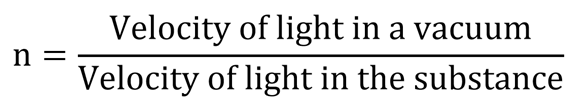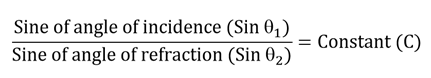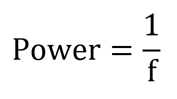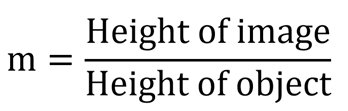# Optics

The branch of physics, which involves the behaviour and properties of light and its interactions with matter including construction of instruments that detect it. It consists of electromagnetic waves that are made up of electric and magnetic fields that travel through space. They vibrate at right angles to each other and to the direction of motion.

The following figure shows the nature of light with vertical axis ‘y’, horizontal axis ‘x’ and the horizontal axis going into the page as ‘z’. The magnetic field oscillates at right angles to the electric field and wave direction. And the electric field oscillates at right angles to the magnetic field and wave direction.### Electromagnetic spectrum

The light waves are a part of electromagnetic radiation including x-rays, ultraviolet rays, infrared rays and radio waves. There are produced by the electron orbit change inside atoms. The range of all possible frequencies of electromagnetic radiation is known as the electromagnetic spectrum.

Some of the common properties of radiation are:

• They travel through space at the light speed of approximately 186,000 miles per second.
• They follow the equation “Velocity (v) = Frequency (f) x Wavelength (λ)”.
• They carry energy from one place to another.

The following figure shows the various forms of electromagnetic spectrum. The spectrum waveform is not proportional to its length throughout.The visible light occurs in the spectrum range between 400nm-700nm, which is visible for a human eye to experience whole range of colours known as visible spectrum.

### Colours

An object appears coloured depending on the absorption and reflection of certain frequencies. The use of optical glass filters will filter out certain wavelengths of various colours and will allow required wavelengths associated with that colour. For example, a red filter allows light with longer wavelengths associated with red to pass through. Some colours may be experienced by scattering of light from surfaces.

Red, green and blue are known as light primary colours. Red, orange, yellow, green, blue and violet are the easily identifiable colours within the visible spectrum. As the frequency gradually changes across the range, the colours blend into each other without any clear boundaries. Black is the absence of any light and white is the combination of all the colours in the visible spectrum.

The colours that are not in the visible spectrum can be formed by combining the colours in the spectrum range. For example, red and blue when mixed together forms purple. Some of the colour combinations are shown below.### Dispersion

The property by which light is spread out based on its colour as it passes through an object by refraction process is known as dispersion. The light energy when shared among the wavelengths in the spectrum results in various colour formation.

Sir Isaac Newton was the first to carry out this study of dispersion using triangular prism. As shown in the following figure, if white light falls onto the prism it will split into spectrum colours.This phenomena occurs when the sun light falls on the water droplets while raining, resulting in the formation of rainbow.

### Reflection

The change in direction of a light wave when it bounces off a boundary is known as reflection, which is often achieved using mirrors. The ray of light known as the incident ray is reflected on the mirror. And the perpendicular line to the mirror at the point the incident ray strikes is called as the normal.The two laws of reflection are as follows.

1. The angle of incidence ‘i’ is equal to the angle of reflection ‘r’.
2. The reflected ray lies in the same place as the incident ray and the normal at the point of incidence.

Based on the laws, the images formed by reflection are easily observed by flat or curved mirrors. The two types of curved mirrors are explained below.

Concave mirror: This has an inward curved surface that is shaped towards the middle and away from the observer.Convex mirror: This has an outward curving surface where the centre is shaped out towards the observer.Point ‘C’ refers to the centre of curvature and point ‘F’ is the principal focal point. In case of concave mirror, the focal point is the point where rays parallel to the principal axis reflect and converge to a point. And in case of convex mirror, they appear to diverge from this point.

### Refraction

The change in direction of the light rays when they move from one medium to another is known as refraction. This direction depends on the density of the medium. The more the medium density the greater the refraction effect.The light ray is bent towards the normal when it enters an optically denser medium at an angle. Thus the angle if refraction ‘r’ is less than the angle of incidence ‘i’ as the light ray moves from air to glass.

The absolute refractive index ‘n’ of a substance is defined as the index of refraction of a substance when a ray passes into it from a vacuum, which is represented as below.The absolute refractive index of a vacuum (nvacuum) and water (nwater) is 1 and 1.33, respectively. In a vacuum, light travels at approximately 300,000km/s, whereas in glass the velocity is around 200,000km/s. The refractive index highly depends on the wavelength of the light.

The two laws of refraction are as follows.

1. The incident ray, the refracted ray and the normal at the point of incidence are all in the same plane.
2. The ratio of the sine of the angle of incidence to the sine of the angle of refraction at the boundary of the two substances is always constant. This law is known as Snell’s law, which is represented as below.### Lenses

The lenses are the most important components in many optical instruments. Concave and convex are the two basic types, which are explained below.

Concave lens: These are diverging lens that are thinnest at its centre, causing the light to spread out once received.Convex lens: These are converging lens that are thickest at its centre, causing the light to bend inwards.Point ‘O’ refers to the optical centre and point ‘F’ is the principal focal point. The distance between the focal point and the optical centre is known as the focal length ‘f’. The ability of the lens to converge or diverge light rays can be calculated using the formula below.The focal length is positive for a convex lens and negative for a concave lens. The linear magnification ‘m’ is calculated using the following formula.